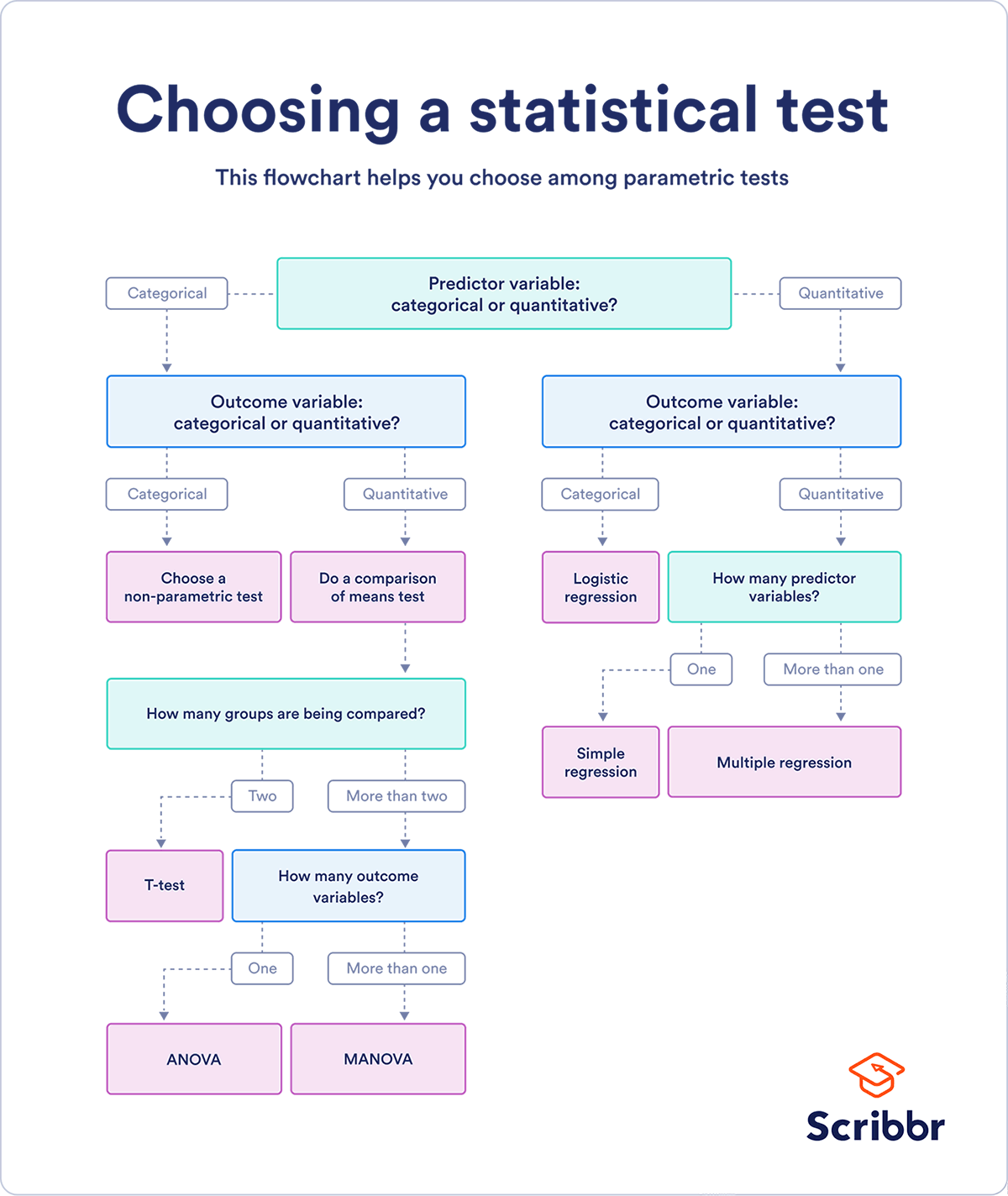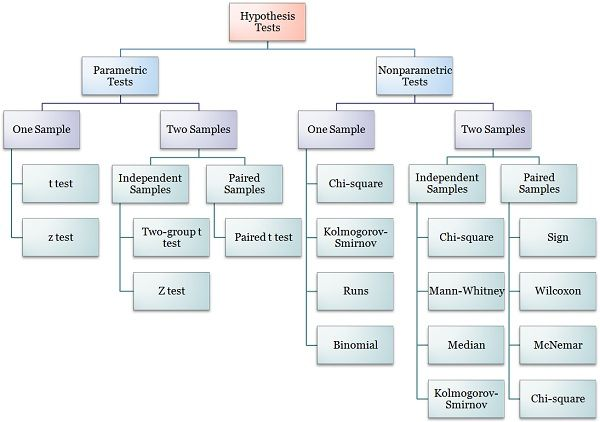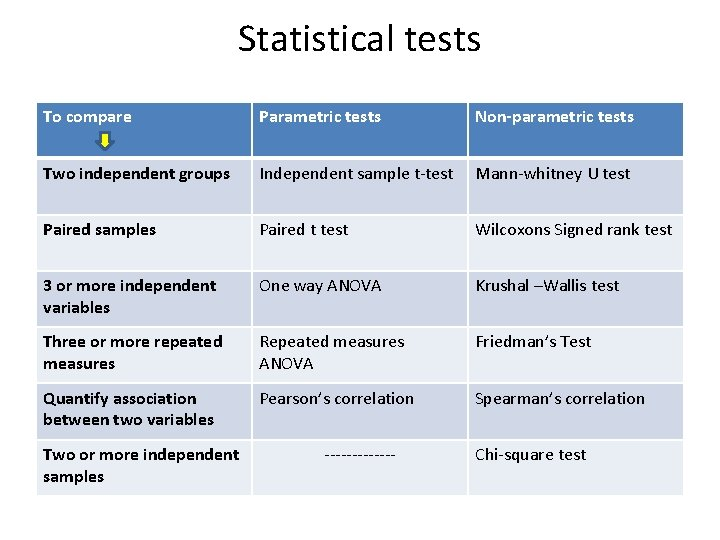All University of Utah libraries course and research guides, in one place.

# Statistical tests

Choosing the appropriate statistical test

## Check out these relevant videos

STATISTICAL TESTS

Statistical analysis is a procedure that allows you to make quantitative assessments about a process or set of processes. The goal is to see if there is enough information to "reject" a theory or hypothesis about how the process works.

Choosing the Correct Statistical Test in SAS, Stata, SPSS and R. (n.d.). Introduction to SAS. UCLA: Statistical Consulting Group. Retrieved May 30, 2022, from https://stats.oarc.ucla.edu/other/mult-pkg/whatstat/

## Parametric tests are statistical tests that assume, among other things, that the data follows a normal distribution. The assumption is that the data of the entire population, not the sample data you're working with, follows a normal distribution.

Use it WHEN

1. The mean represents the center of data distribution

2. The Sample size is large

3. The data is normally distributed

Data that is assumed to have a normal distribution can be analyzed using the following statistical analyses:

• t-tests

• ANOVA

• Regression Analysis

• Correlation CoefficientBevans, R. (2022, May 6). Statistical tests: which one should you use? Scribbr. https://www.scribbr.com/statistics/statistical-tests/

### NON-PARAMETRIC TESTS

Nonparametric tests, often known as distribution-free tests, are statistical tests that make no assumptions about the data's distribution. Nonparametric tests are based on ordinal variables or the rank of values in the data.

Use it WHEN

1. The median represents the center of data distribution

2. The Sample size is large or small

3. The data is not normally distributed

Data that is assumed to not have a normal distribution can be analyzed using the following statistical analyses:

• 1-sample sign test

• 1-sample Wilcoxon Signed Rank test

• Friedman test

• Kruskal-Wallis test

• Mann-Whitney test

• Mood’s Median test

• Spearman Rank CorrelationS, S. (2017, September 1). Difference Between Parametric and Nonparametric Test (with Comparison Chart). Key Differences. https://keydifferences.com/difference-between-parametric-and-nonparametric-test.html

Each parametric test has its equivalent non-parametric test that is given in the below imageInferential statistics. (n.d.). Slidetodoc.Com. https://slidetodoc.com/inferential-statistics-introduction-descriptive-statistics-judgmentsinferences-related-to/